﻿ 实用Excel技巧分享：16000行数据自动分组编号 - 自由资讯

# 实用Excel技巧分享：16000行数据自动分组编号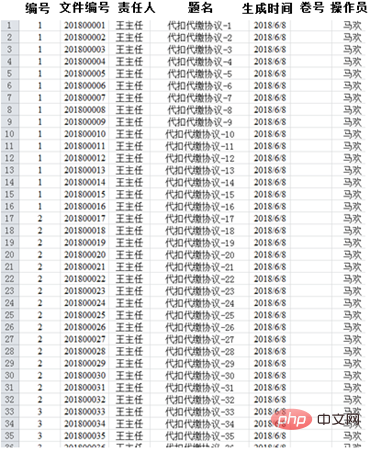1、操作步骤

（1）编辑“连续重复编号”公式。在A1单元格中输入公式：=IF(MOD(ROW(A1),16)=0,ROW(A1)/16,INT(ROW(A1)/16)+1)。如下图所示：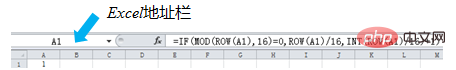（2）快速选定“连续重复编号”区域。用鼠标选点击A1单元格；在Excel地址栏输入A16000; 按住“Shift” 键不松手，再按“Enter”键。完成以上三个步骤之后就可以快速选定需要连续重复编号的区域。如下图所示：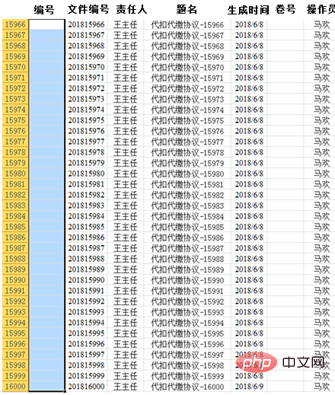（3）快速填充公式。选定“连续重复编号”区域之后，在“开始”选项卡中，点击“填充”选项卡，选择“向下”选项，即可完成公式自动填充。“连续重复编号”结果如下图所示：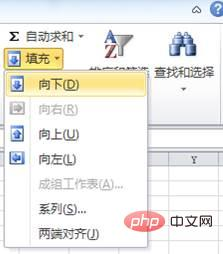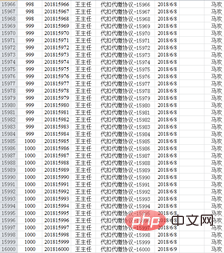2、函数解释

• ROW()函数。ROW()函数返回该行任意一个单元格所在的行号，如：ROW(A13)=13、 ROW(B13)=13。

• INT()函数。取整函数，如：INT (0.1)=0、INT (2)=2、INT (3.7)=3、INT(-1.1)=-2。即：当x≥0时，INT (x)= x值的整数部分（非四舍五入）；

当x<0时，INT (x)=（x值整数部分的绝对值+1）乘以-1。

• MOD()函数。求两个数相除的余数，如：MOD(1,16)=1、MOD(16,16)=0。当MOD(x,y)=0时，x即为y的整数倍。（注：第一个参数为被除数，第二个参数为除数）

• IF()函数。IF()函数有三个参数，即：IF(逻辑判断表达式,结果1,结果2)，当逻辑判断表达式成立（即为真：TRUE），IF()函数返回结果1；当逻辑判断表达式不成立（即为假：FALSE），IF()函数返回结果2。

`=IF(MOD(ROW(A1),16)=0,ROW(A1)/16,INT(ROW(A1)/16)+1)`

IF第一参数MOD(ROW(A1),16)=0：判断单元格所在行号除以16后的余数是否等于0，也就是行号是否能被16整除。很显然，16、32等能被16整除，余数=0，条件成立；15、17等不能被16整除，余数≠0，条件不成立。

IF第二参数ROW(A1)/16：当第一参数条件成立时，编号等于行号除以16的商。例如：

A16，编号= ROW(A16)/16=16/16=1

A32，编号= ROW(A32)/16=32/16=2

……

IF 第三参数INT(ROW(A1)/16)+1：当第一参数不成立时，编号等于行号除以16的商取整后再加1。例如：

A15，编号= INT(ROW(A15)/16)+1= INT（15/16）+1=INT( 0.9375) +1=0+1=1

A17，编号= INT(ROW(A17)/16)+1= INT（17/16）+1=INT( 1.0625) +1=1+1=2

……

1、操作步骤

（1）进入VBA编辑窗口。按组合键Alt+F11（或者单击“开发工具”选项卡“Visual Basic”按钮）进入Excel中的Visual Basic。

（2）在“插入”菜单中选择“模块”命令，然后在右侧窗口中输入以下代码：

```Sub rep()
Dim i%
For i = 1 To 1000
Sheet2.Range("A" & (16 * i - 15) & ":A" & (16 * i)) = i
Next i
End Sub```

（3）按F5键（或者单击快速工具栏中的运行按钮）运行以上程序之后，即可在A1：A16000单元格中快速生成连续重复编号，运算过程不到一秒钟，如下图所示。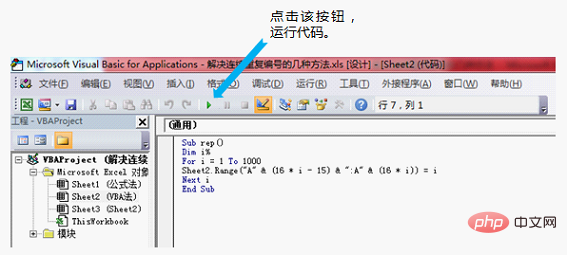2、代码解释

`For i = 1 To 1000`：用于指定编号的取值范围。如果编号取值是2到25，则写成`For i = 2 To 25`

Sheet2：用于指定需要编号的工作表。sheet2并非指的是工作表的名称，而是指Excel工作簿的第2张表（从左向右），若需要在第1张表中生成编号，只需将代码改成sheet1即可，其他情况类推。

`Range("A" & (16 * i - 15) & ":A" & (16 * i))`：用于指定编号的单元格范围和规则，意思是从A1单元格开始到`A（16 * i）`单元格止每16个单元格编1个号。

"A"指的是需要生产编号的列号，若需要在B列或C列生成编号，则写成“B”或者“C”；

• 快速选定某区域。用鼠标选定备选区域左上角的单元格（如：A1）；在Excel地址栏输入备选区域右下角的单元格（如：B16）; 按住“Shift” 键不松手，再按“Enter”键。完成以上三个步骤之后即可快速选定某区域。

• 巧用Excel函数是关键。有很多初学者已经掌握了大量的Excel基本函数，但是就是不知道怎么样、何时用、用哪种。我建议大家把Excel基本函数当作我们炒菜的“素材”，把数学规律、事件内隐藏的逻辑关系当作炒菜的“工具”，多思考、勤实践，那么再遇到问题，就会“信手捏来”了。﻿